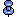# Using bcp to correct partition balance

If you need to load additional data into a partitioned table that does not have clustered indexes, and sp_helpartition shows that some partitions contain many more pages than others, you can use the bulk copy session to help balance number of rows on each partition.

The following example shows that the table has only 487 pages on one partition, and 917 on another:

```partitionid firstpage   controlpage ptn_data_pages
----------- ----------- ----------- --------------
1      189825      189824            812
2      204601      204600            487
3      189689      189688            917

(3 rows affected)
Partitions Average Pages Maximum Pages Minimum Pages Ratio (Max/Avg)
---------- ------------- ------------- ------------- ---------------
3           738           917           487        1.242547
```

The number of rows to add to each partition can be computed by:

• Determining the average number of rows that would be in each partition if they were evenly balanced, that is, the sum of the current rows and the rows to be added, divided by the number of partitions

• Estimating the current number of rows on each partition, and subtracting that from the target average

The formula can be summarized as:

```Rows to add = (total_old_rows + total_new_rows)/#_of_partitions
- rows_in_this_partition
```

This sample procedure uses values stored in systabstats and syspartitions to perform the calculations:

```create procedure help_skew @object_name varchar(30), @newrows int
as
declare @rows int, @pages int, @rowsperpage int,
@num_parts int
select @rows = rowcnt, @pages = pagecnt
from systabstats
where id = object_id(@object_name) and indid in (0,1)
select @rowsperpage = floor(@rows/@pages)
select @num_parts = count(*) from syspartitions
where id = object_id(@object_name)

select partitionid,  (@rows + @newrows)/@num_parts -
from syspartitions
where id = object_id (@object_name)
```

Use this procedure to determine how many rows to add to each partition in the customer table, such as when 18,000 rows need to be copied in. The results are shown below the syntax.

```help_skew customer, 18000
```
```partitionid rows_to_add------------------
1        5255
2        9155
3        3995
```If the partition skew is large, and the number of rows to be added is small, this procedure returns negative numbers for those rows that contain more than the average number of final rows.

Query results are more accurate if you run update statistics and update partition statistics so that table and partition statistics are current.

With the results from help_skew, you can then split the file containing the data to be loaded into separate files of that length, or use the -F (first) and -L (last) flags to bcp.